# Kraken Daily Market Report for June 10 2020

## Overview

• Total trading volume up slightly from yesterday at $156 million. A little after 18:00 UTC, Bitcoin and Ethereum prices jumped up 3%, and then retreated 2%. This caused a short spike in trading volume. • Yesterday’s best performer, NANO, was also today’s biggest loser. However, the losses of 1.5% were only a fraction of yesterday’s 15% gains (all over USD). Today’s best performers were Siacoin (SC) and Melon (MLN), both with 13% returns over USD. • Some new pairs came out today, check out the the Spread % table to see the spreads of all traded pairs. June 10, 2020$152.5M traded across all markets today
Crypto, EUR, USD, JPY, CAD, GBP, CHF
XBT
$9892.4 ↑1.2%$107.3M
ETH
$247.94 ↑1.6%$20.3M
USDT
$1.0004 ↓0.01%$10.9M
$0.0835 ↑0.7%$3.49M
BCH
$257.19 ↑1.3%$2.15M
XRP
$0.2027 ↑0.7%$2.0M
XTZ
$2.8899 ↓0.5%$1.45M
XMR
$69.75 ↑2.5%$1.34M
USDC
$0.9998 ↑0.01%$1.28M
LTC
$46.704 ↑1.8%$1.12M
NANO
$1.1781 ↓1.5%$1.05M
$4.4221 ↓0.4%$966K
REP
$15.744 ↑6.3%$551K
DAI
$1.0048 ↑0.17%$545K
ICX
$0.3619 ↑8.5%$424K
SC
$0.0029 ↑13%$419K
XLM
$0.0788 ↑0.8%$378K
WAVES
$1.2444 ↑6.2%$371K
ZEC
$55.260 ↑6.3%$362K
EOS
$2.7592 ↑0.19%$333K
PAXG
$1732.3 ↑0.9%$280K
ATOM
$3.1172 ↑0.07%$269K
BAT
$0.2505 ↓0.4%$261K
ALGO
$0.2472 ↑1.9%$207K
DASH
$78.280 ↑1.0%$166K
MLN
$4.7129 ↑13%$149K
OMG
$1.6497 ↑0.03%$140K
OXT
$0.1939 ↓0.08%$128K
QTUM
$1.8631 ↑3.1%$111K
ETC
$6.8157 ↑0.5%$105K
TRX
$0.0176 ↑0.27%$102K
LSK
$1.2998 ↓0.23%$68.9K
GNO
$31.385 ↑2.7%$40.8K
XDG
$0.0025 ↑0.7%$31.4K

#####################. Trading Volume by Asset. ##########################################

The figures below break down the trading volume of the largest, mid-size, and smallest assets. Cryptos are in purple, fiats are in blue. For each asset, the chart contains the daily trading volume in USD, and the percentage of the total trading volume. The percentages for fiats and cryptos are treated separately, so that they both add up to 100%.

Figure 1: Largest trading assets: trading volume (measured in USD) and its percentage of the total trading volume (June 10 2020)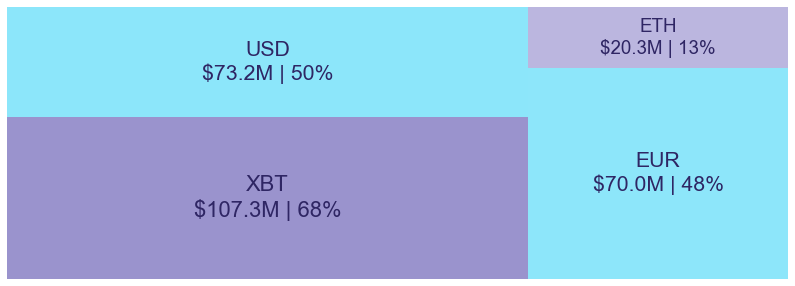Figure 2: Mid-size trading assets: (measured in USD) (June 10 2020)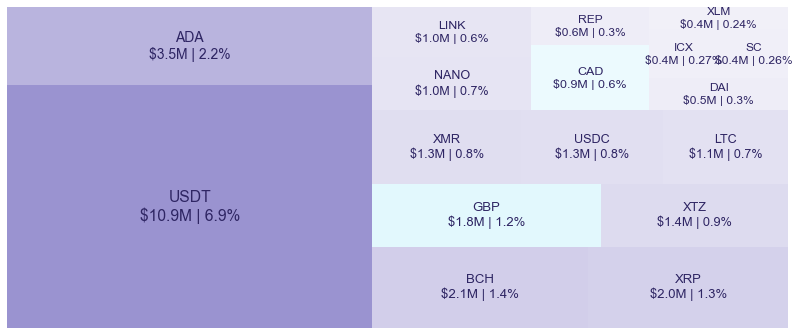Figure 3: Smallest trading assets: (measured in USD) (June 10 2020)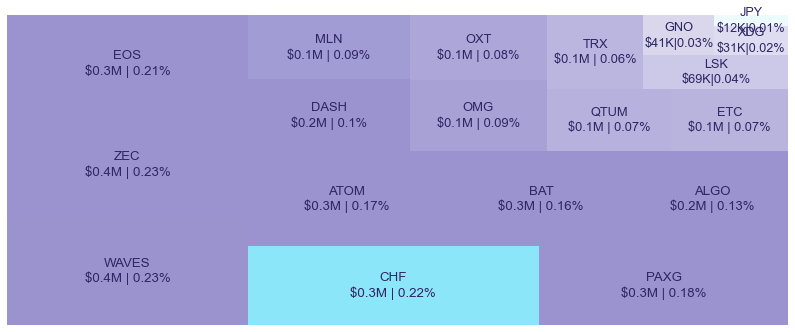Spread percentage is the width of the bid/ask spread divided by the bid/ask midpoint. The values are generated by taking the median spread percentage over each minute, then the average of the medians over the day.

Figure 4: Average spread % by pair (June 10 2020)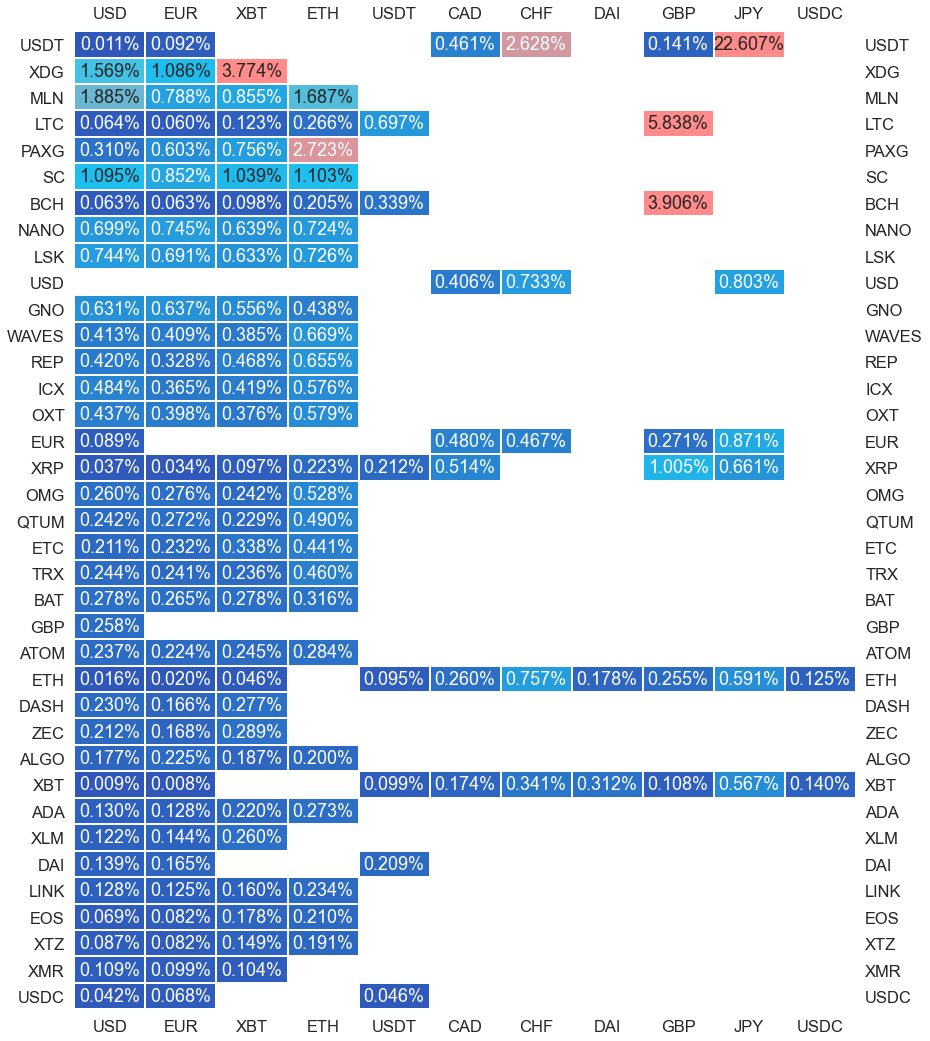.

#########. Returns and Volume ############################################

## Returns and Volume

Figure 5: Returns of the four highest volume pairs (June 10 2020)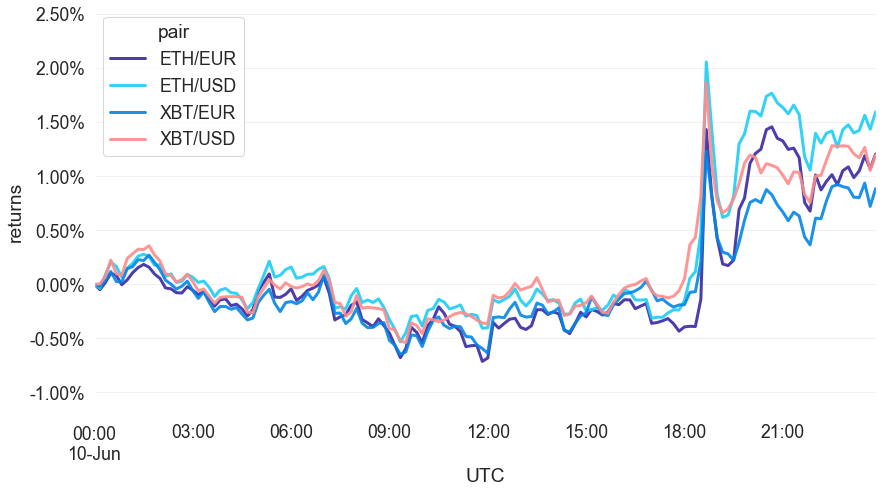Figure 6: Volume of the major currencies and an average line that fits the data to a sinusoidal curve to show the daily volume highs and lows (June 10 2020)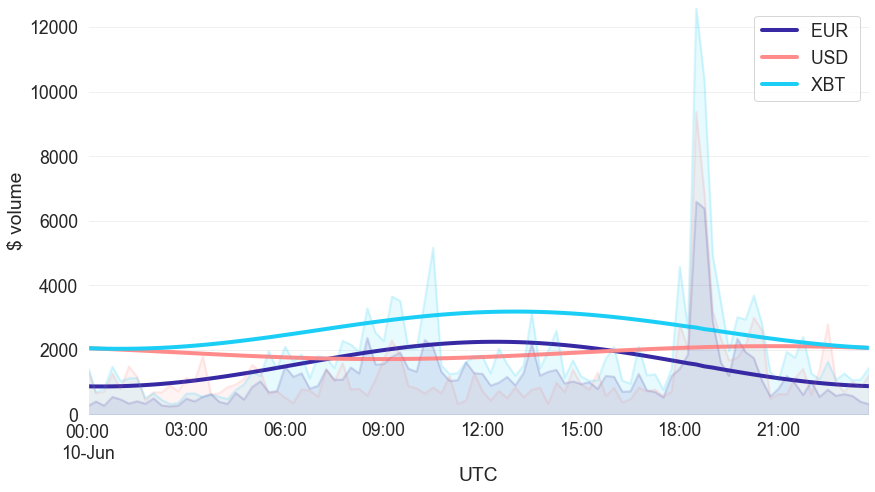###########. Daily Returns. #################################################

## Daily Returns %

Figure 7: Returns over USD and XBT. Relative volume is indicated by the size of the font. (June 10 2020)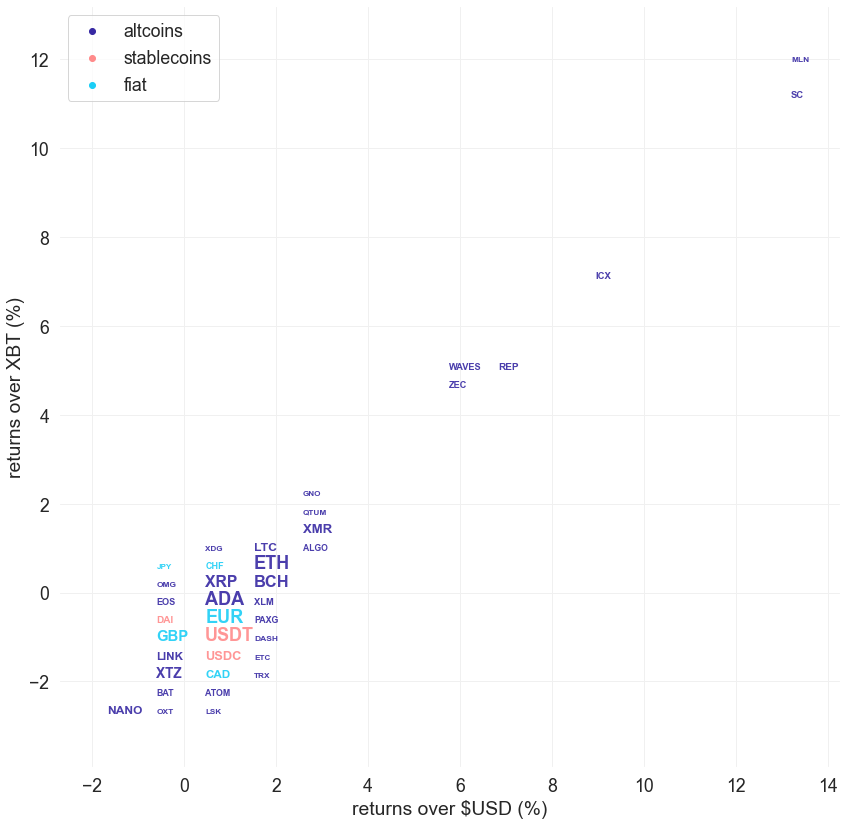###########. Disclaimer #################################################

The values generated in this report are from public market data distributed from Kraken WebSockets api. The total volumes and returns are calculated over the reporting day using UTC time.